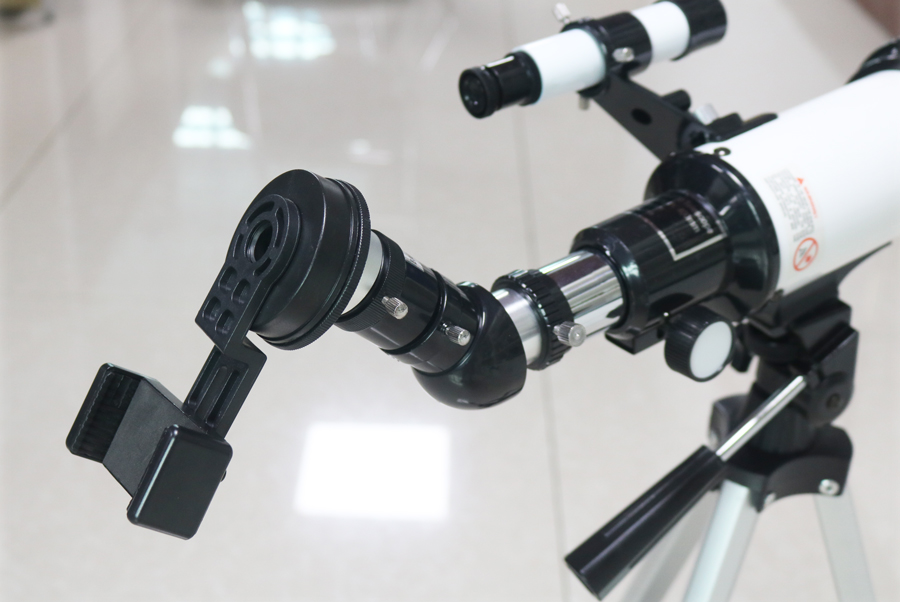# How to adjust the sight distance of the telescopeFirst, adjust the positive and negative diopter scales of the left and right eyepieces of the telescope to 0 scale. Hold the left and right mirror bodies of the telescope with both hands, and search for distant targets while stretching or pressing the left and right mirror bodies to make the eye distance of the telescope the same as the interpupillary distance of the human eye, stop adjusting.

Then, after searching for the target and locking the target, turn the left eyepiece diopter handwheel to make the target image and the division image of the left branch of the telescope completely clear, and then turn the right eyepiece diopter handwheel to make the target image of the right system completely clear , Then the adjustment of the observed target is completed. Because the optical path of the telescope is designed with a dynamic auto-focusing function, after the telescope’s clarity is adjusted, it is not necessary to refocus when observing targets at different distances again.

Then, the direction angle refers to the angle between the two targets (or two ends of a target in the horizontal direction) to the telescope on the horizontal plane.

a) When the directional angle of the two targets is smaller than the reticle range of the direction angle measurement in the telescope, align the reticle on one end of the reticle to the target (target 1), and then watch the other target (target 2) align with the reticle of the reticle The value is the density of the measured direction angle.

b) When the direction angle of the two targets is greater than the direction angle division in the telescope, any target (target 3) between the two targets (target 1, 2) can be used for segmental measurement. The sum of the measured values of the segments is the measured direction angle, and the measured direction angle is 1-10 (110 density).

Then, the angle between any two targets (or two ends of a target) on the vertical plane of the telescope is called the high and low angle.

a) When the high and low intersection angle of the target is relatively small, align the cross center of the reticle (or any reticle) below the target, and see the reticle value between the corresponding reticle above the target, which is the measured height and low angle As shown in the figure, the angle of the target is 0-15 (15 dense). To

b) When the high and low included angle of the target is relatively large, the method of segmented measurement can be used, and the value of the segmented measurement is added together, which is the high and low included angle

a) When the target height is 2m, the lower end of the target is aligned with the horizontal line of the line-of-sight division, and the reading at the point where the upper end of the target is tangent to the line-of-sight division is the distance between the target and the observer, as shown in the figure, the target The distance to the observer is 550m.

b) When the height of the target is greater than (or less than) 2m, the actual distance is calculated as follows: L=L1xH/2(m)

Finally, when the ambient light is dim or when observing at night, it is recommended to use a telescope with an exit pupil diameter of 7mm or more. Because the pupil diameter of the human eye is about 2-3mm in the daytime and about 6-7mm in the dark, the telescope can collect more light than the naked eye